### Home > A2C > Chapter Ch11 > Lesson 11.3.1 > Problem11-134

11-134.
1. A small rocket is launched from five meters below ground level reaches a height of 3 meters above the ground after 4 seconds. On the way down it is 3 meters above the ground after 8 seconds. Homework Help ✎

1. What are three data points?

2. Draw a rough sketch of the height of the rocket over time.

3. Find the equation of the parabola based on the data.

4. When will the rocket hit the ground?

5. What is the domain for this function?

6. For what part of the domain is the rocket below ground?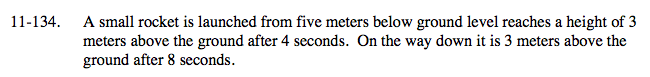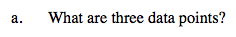Define the variables; x = time, y = height.

(0, −5), (4, 3), (8, 3)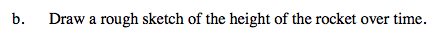See part (a).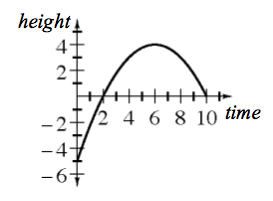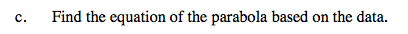Use the points from (a) and the general equation y = ax2 + bx + c.

$y=-\frac{1}{4}x^{2}+3x-5$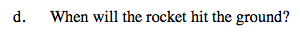See the graph from part (b).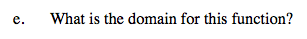See the graph from part (b).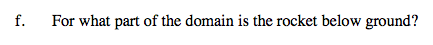See the graph from part (b).